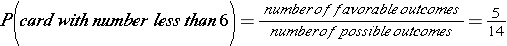# Theoretical Probability

In this lesson, you will learn to find the theoretical probability of an event. Theoretical probability is used to find the probability of an event when all the outcomes are equally likely. The theoretical probability of an event is the ratio of the number of ways the event can occur to the number of possible outcomes.### ExampleShelby is going to select a random card from a stack of 14 cards. They are numbered from 1 to 14. What is the probability that she chose a card with a number less than 6?

1. Count the number of favorable outcomes. There are 5 cards less than 6 (1, 2, 3, 4, and 5)

2. Count the number of possible outcomes. There are a total of 14 cards, each with the same chance of being drawn

3. Find the theoretical probability.

4.5. The probability of selecting a card less than 6 isand is unlikely.

### ExampleAndy has 25 marbles in a bag. Of these, 10 are clear and 15 are blue. Find the probability of drawing a clear marble from the bag? Write your answer as a fraction, as a decimal, and as a percent.

1.2.3. The theoretical probability of drawing a clear marble is two fifths, forty percent, and forty hundredths.

We have learned that theoretical probability is what we expect to happen when all outcomes are equally likely, whereas experimental probability is what really happens when we actually do the experiment. We calculate probability the same and the more trials we conduct, the closer experimental probability gets to theoretical probability.

Watch the video to see examples and comparisons of experimental and theoretical probability.

Now see how well you understand the terms involved in Theoretical Probability.

## Theoretical Probability Practice

What fills the blank?

A. probability  B. trials  C. favorable  D. theoretical  E. fair  F. fraction  G. percent  H. one  I. experimental  J. decimal

Match the items.

1.  ______ probability is used to calculate the probability of an event when all outcomes are equally likely.
2. You can write a ______ as a decimal or a fraction.
3. If each possible outcome of an experiment is equally likely, the experiment is said to be ______.
4. You can write a __________ as a fraction or a percent.
5. A ___________ outcome is one that you are looking for when you conduct an experiment.
6. ______ probability compares the number of times an event occurs to the total number of trials.
7. You can write ______ as a fraction, a decimal, or a percent.
8. You can write a ______ as a decimal or as a percent.
9. The sum of the probabilities of an event and its complement is _____.
10. In experimental probability, the more _______ you have, the more accurate the estimate is likely to be.